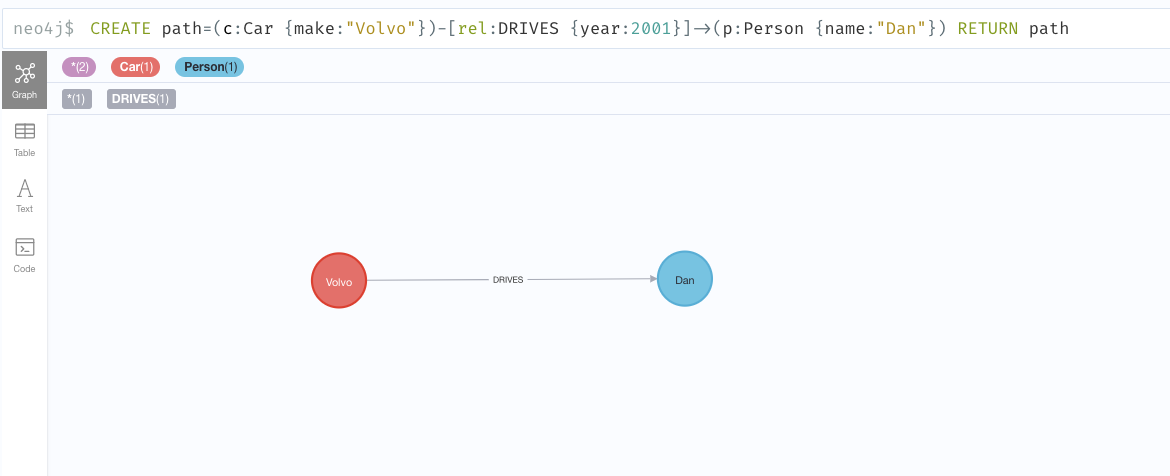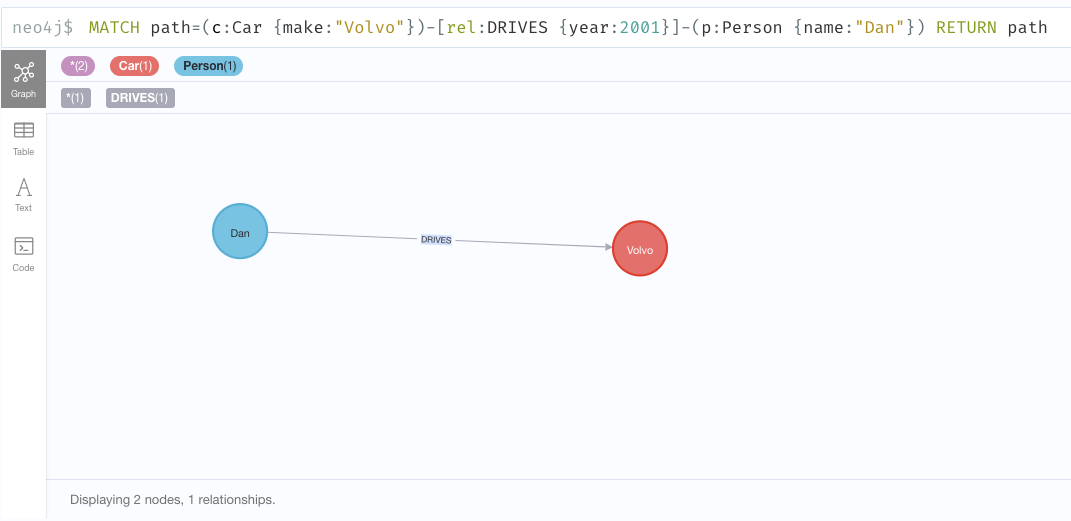# Invert relationship

The available procedure is described in the table below:

 `call apoc.refactor.invert(rel)` inverts relationship direction

## Example Usage

The example below will help us learn how to use this procedure.

The following creates a graph containing two nodes connected by a relationship:
``CREATE path=(c:Car {make:"Volvo"})-[rel:DRIVES {year:2001}]->(p:Person {name:"Dan"}) RETURN path``The following inverts the direction of the relationship:
``````MATCH (c:Car)-[rel:DRIVES]->(p:Person)
CALL apoc.refactor.invert(rel)
yield input, output
RETURN input, output``````
Table 1. Results
input output

2

{"identity":3,"start":9,"end":8,"type":"DRIVES","properties":{"year":2001}}

If we execute `MATCH path=(c:Car {make:"Volvo"})-[rel:DRIVES {year:2001}]-(p:Person {name:"Dan"}) RETURN path`, it will result in the following graph: